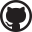# Dynamic Epistemic Logic Playground

Current agent:
Number of propositional variables:
No world selected
 p: q: r: s: t:
Enter a formula: or
• When entering a formula:
• use ~A for $\lnot{}A$
• use (A & B) for $(A\land{}B)$
• use (A | B) for $(A\lor{}B)$
• use (A -> B) for $(A\rightarrow{}B)$
• use K{a,b,c}A for $K_{a,b,c}A$
• use [A]B for $[A]B$

## Editing Instructions

• Click in the open space to add a world
• Drag between worlds to add a transition for the current agent
• Ctrl-drag a world to move graph layout
• Click a world or a transition to select it
• When a world is selected:
• R toggles reflexivity for the current agent
• Delete removes the world
• When a transition is selected:
• L(eft), R(ight), B(oth) change direction
• Delete removes the transition
• Copy the URL from your browser's URL bar to share your model!

## What is (Epistemic) Logic?

Epistemic Logic is way of formally reasoning about knowledge. In order to understand how Epistemic logic works, let's start with classical logic and build up from there.

### Classical Logic and Formulas

In classical logic we start with propositional variables, our propositional variables are $p$, $q$, $r$, $s$, and $t$. Each propositional variable can either be true or false. For example we can say $p$ is false, written as $\lnot p$, and let's say $q$ is true, written just as $q$.

Alongside propositional variables, we have the classical logical connectives:

• $\lnot A$ (not) which is true when $A$ is false,
• $A \land B$ (and) which is true when both $A$ and $B$ are true,
• $A \lor B$ (or) which is true when $A$ is true, or $B$ is true, or both are true,
• and $A \rightarrow B$ (conditional) which is true if $B$ is true whenever $A$ is true.
We can apply the logical connectives, along with parantheses, to propositional variables to build up complex formulas. For example $(p \rightarrow q) \land (q \rightarrow p)$ is a formula which is true when $p = q$, or $p \lor \lnot p$ is a formula which is always true!
pro tip: $(p \rightarrow q) \land (q \rightarrow p)$ can be written shorthand as (p <-> q) in the playground.

### Worlds, Agents, and Epistemic Logic

Epistemic Logic is designed for reasoning about the knowledge of someone (we call that someone an agent). We might imagine that an agent does not know whether they are in a situation where $p$ is true, or a situation where $p$ is not true, i.e. $\lnot p$. We express situations as worlds. We express an agent's knowledge by drawing relations (arrows) between worlds. These worlds and agent relations together are called a model.

Here's an example model for when an agent $a$ doesn't know if they are in a situation where $p$, or a situation where $\lnot p$:

When you enter a classical formula into the playground, the formula is evaluated at each world as if that world was just a classical logic by itself. Epistemic logic introduces a new logical connective which takes advantage of agents' relations between worlds:

• $K_b A$ (knowledge of an agent $b$) which is true at a world when $A$ is true at all worlds connected to that world by $b$ arrows.
• along with $K_{a,b,c} A$ which is shorthand for $K_a A\land K_b A\land K_c A$.
Now, I understand if this definition isn't intuitive, it's not obvious what $K_b A$ has to do with knowledge. In order to start intuitively understanding, let's consider our previous example. We can write a formula to check whether an agent $a$ knows that they are in a situation where $p$ or that they know they are in a situation where $\lnot p$: This formula is false, by definition of $K_a$, at both worlds because they are each connected to both a world where $p$ is false and a world where $p$ is true. In an intuitive sense, agent $a$ does not know what $p$ is because they cannot distinguish between the world where $p$ is true and the world where $p$ is false.

If you add more than one agent you can reason about one agent's knowledge of another agent's knowledge e.g. $K_a K_b p$, $a$ knows that $b$ knows that $p$ is true.

Finally, for the sake of representing knowledge, in Epistemic logic it is expected that all agent's relations between worlds are equivalence relations, i.e. each agent's relation is:

• reflexive, so each world is related to itself;
• symmetric, if a world $0$ is related to a world $1$, then world $1$ is also related to world $0$;
• and transitive, if a world $0$ is related to a world $1$, and world $1$ is related to world $2$ then world $0$ has to be related to world $2$ as well.

### Dynamic Epistemic Logic - Public Announcement

Dynamic Epistemic Logic introduces announcement, a mechanism for publicly announcing facts that become the knowledge of all agents. Given a formula $A$, a public announcement removes all worlds where $A$ is not true.

Dynamic Epistemic Logic also introduces a logical connective:

• $[A]B$ (public announcement of $A$) which is true at a world if $B$ is true after $A$ is announced, or if the world is removed by the announcement.
Try out the announcement button and the announcement connective in the playground.

## Project Information

This project is based on the open-source Modal Logic Playground by Ross Kirsling. The Epistemic Logic Playground was made by Elliot Evans. Special thanks to my non-classical logic instructors Richard Zach and Audrey Yap, as well as my classmates Jaxson, Parker, Koray, Alejandro, and Tina who provided feedback on this project.

More resources on Epistemic Logic and this project: